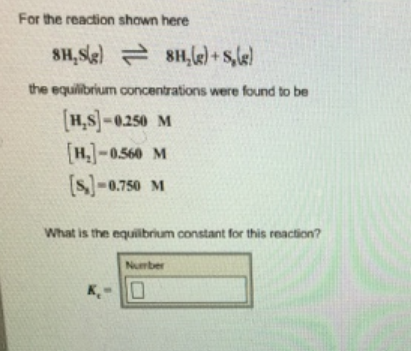# Problem: For the reaction shown here8 H2S (g) ⇌ 8 H2(g) + S8(g)the equilibrium concentrations were found to be[H2S] = 0.250 M[H2] = 0.560 M[S8] = 0.750 MWhat is the equilibrium constant for this reaction?

###### FREE Expert Solution
95% (155 ratings)###### Problem Details

For the reaction shown here
8 H2S (g) ⇌ 8 H2(g) + S8(g)
the equilibrium concentrations were found to be
[H2S] = 0.250 M
[H2] = 0.560 M
[S8] = 0.750 M

What is the equilibrium constant for this reaction?